# Icosidodecahedron

Icosidodecahedron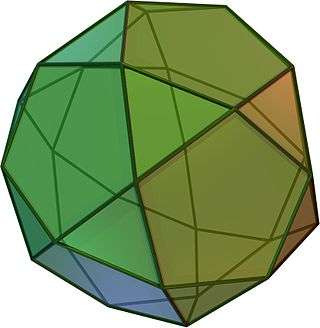TypeArchimedean solid
Uniform polyhedron
ElementsF = 32, E = 60, V = 30 (χ = 2)
Faces by sides20{3}+12{5}
Schläfli symbolsr{5,3}
t1{5,3}
Wythoff symbol2 | 3 5
Coxeter diagramSymmetry groupIh, H3, [5,3], (*532), order 120
Rotation groupI, [5,3]+, (532), order 60
Dihedral Angle142.62°ReferencesU24, C28, W12
PropertiesSemiregular convex quasiregular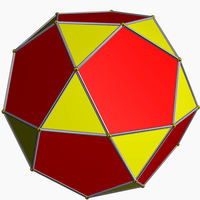Colored faces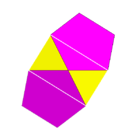3.5.3.5
(Vertex figure)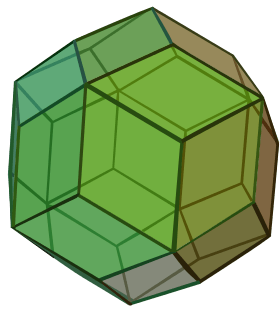Rhombic triacontahedron
(dual polyhedron)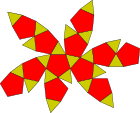Net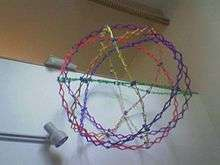A Hoberman sphere as an icosidodecahedron

In geometry, an icosidodecahedron is a polyhedron with twenty triangular faces and twelve pentagonal faces. An icosidodecahedron has 30 identical vertices, with two triangles and two pentagons meeting at each, and 60 identical edges, each separating a triangle from a pentagon. As such it is one of the Archimedean solids and more particularly, a quasiregular polyhedron.

An icosidodecahedron has icosahedral symmetry, and its first stellation is the compound of a dodecahedron and its dual icosahedron, with the vertices of the icosahedron located at the midpoints of the edges of either.

Its dual polyhedron is the rhombic triacontahedron. An icosidodecahedron can be split along any of six planes to form a pair of pentagonal rotundae, which belong among the Johnson solids.

The icosidodecahedron can be considered a pentagonal gyrobirotunda, as a combination of two rotundae (compare pentagonal orthobirotunda, one of the Johnson solids). In this form its symmetry is D5d, [10,2+], (2*5), order 20.

The wire-frame figure of the icosidodecahedron consists of six flat regular decagons, meeting in pairs at each of the 30 vertices.

## Cartesian coordinates

Convenient Cartesian coordinates for the vertices of an icosidodecahedron with unit edges are given by the even permutations of:

• (0, 0, ±φ)
• 1/2, ±φ/2, ±1 + φ/2)

where φ is the golden ratio, 1 + 5/2.

## Orthogonal projections

The icosidodecahedron has four special orthogonal projections, centered on a vertex, an edge, a triangular face, and a pentagonal face. The last two correspond to the A2 and H2 Coxeter planes.

Orthogonal projections
Centered by Vertex Edge Face
Triangle
Face
Pentagon
Image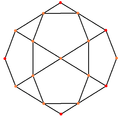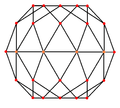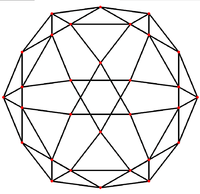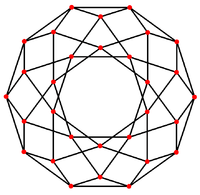Projective
symmetry
   
Dual
image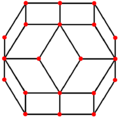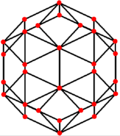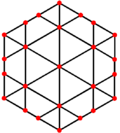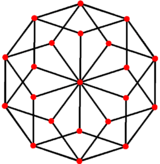## Surface area and volume

The surface area A and the volume V of the icosidodecahedron of edge length a are:## Spherical tiling

The icosidodecahedron can also be represented as a spherical tiling, and projected onto the plane via a stereographic projection. This projection is conformal, preserving angles but not areas or lengths. Straight lines on the sphere are projected as circular arcs on the plane.

 Orthographic projection Stereographic projections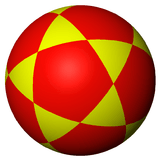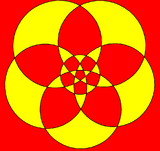Pentagon-centered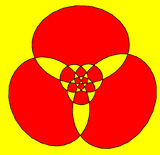Triangle-centered

The icosidodecahedron is a rectified dodecahedron and also a rectified icosahedron, existing as the full-edge truncation between these regular solids.

The icosidodecahedron contains 12 pentagons of the dodecahedron and 20 triangles of the icosahedron:

The icosidodecahedron exists in a sequence of symmetries of quasiregular polyhedra and tilings with vertex configurations (3.n)2, progressing from tilings of the sphere to the Euclidean plane and into the hyperbolic plane. With orbifold notation symmetry of *n32 all of these tilings are wythoff construction within a fundamental domain of symmetry, with generator points at the right angle corner of the domain.

### Dissection

The icosidodecahedron is related to the Johnson solid called a pentagonal orthobirotunda created by two pentagonal rotunda connected as mirror images. The icosidodecahedron can therefore be called a pentagonal gyrobirotunda with the gyration between top and bottom halves.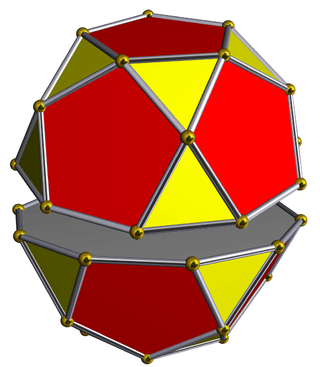(Dissection)Icosidodecahedron(pentagonal gyrobirotunda)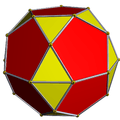Pentagonal orthobirotunda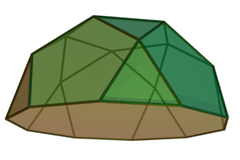Pentagonal rotunda

Eight uniform star polyhedra share the same vertex arrangement. Of these, two also share the same edge arrangement: the small icosihemidodecahedron (having the triangular faces in common), and the small dodecahemidodecahedron (having the pentagonal faces in common). The vertex arrangement is also shared with the compounds of five octahedra and of five tetrahemihexahedra.Icosidodecahedron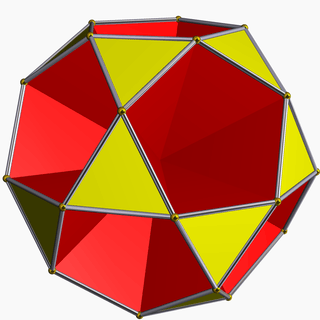Small icosihemidodecahedron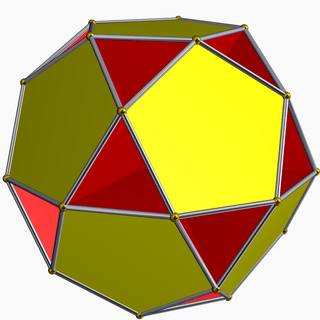Small dodecahemidodecahedron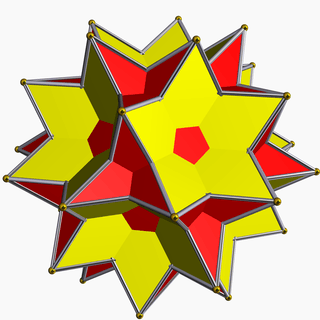Great icosidodecahedron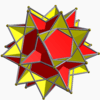Great dodecahemidodecahedron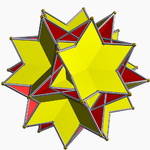Great icosihemidodecahedron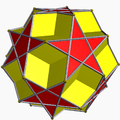Dodecadodecahedron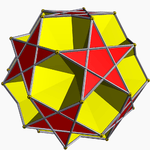Small dodecahemicosahedron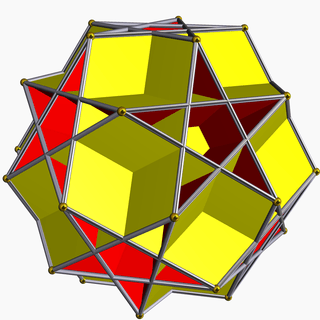Great dodecahemicosahedron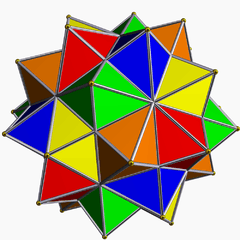Compound of five octahedra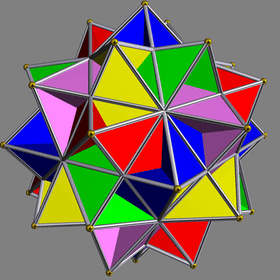Compound of five tetrahemihexahedra

In four-dimensional geometry the icosidodecahedron appears in the regular 600-cell as the equatorial slice that belongs to the vertex-first passage of the 600-cell through 3D space. In other words: the 30 vertices of the 600-cell which lie at arc distances of 90 degrees on its circumscribed hypersphere from a pair of opposite vertices, are the vertices of an icosidodecahedron. The wire frame figure of the 600-cell consists of 72 flat regular decagons. Six of these are the equatorial decagons to a pair of opposite vertices. They are precisely the six decagons which form the wire frame figure of the icosidodecahedron.

## Icosidodecahedral graph

Icosidodecahedral graph5-fold symmetry Schlegel diagram
Vertices 30
Edges 60
Automorphisms 120
Properties Quartic graph, Hamiltonian, regular

In the mathematical field of graph theory, a icosidodecahedral graph is the graph of vertices and edges of the icosidodecahedron, one of the Archimedean solids. It has 30 vertices and 60 edges, and is a quartic graph Archimedean graph.6-fold symmetry10-fold symmetry